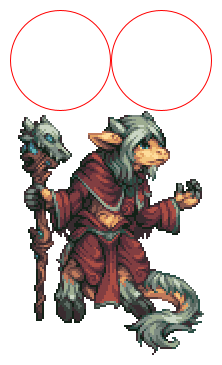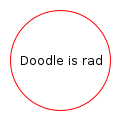# Using Algebras

This section gives recipes for using Doodle’s algebras, either to target one backend or to work across multiple backends.

## What Are Algebras?

Each algebra defines an interface for something related to creating pictures. For example, the `Layout` algebra defines some basic methods for positioning pictures while the `Style` algebra defines methods for changing the fill and stroke of a picture. All algebras extend the `Algebra` base trait, which requires an algebra declare some type that indicates the type of value returned by calling methods on the algebra.

Tagless final style allows for a lot of flexibility. Back ends only implement the algebras that they support, which means that different backends can support different features. This allows us to access the full capabilities of each backend (assuming that someone has taken the time to write an algebra for the features you are interested in!)

## Using Backend Specific Features

Use the following recipe to write code using backend specific features (for example, the `Bitmap` algebra which is currently only supported by the `Java 2D` backend).

The first step is to import the backend and syntax extensions, some Cats implicits we’ll need, and the Cats Effect runtime. We’ll use `java2d` as our backend.

``````import doodle.java2d._
import doodle.syntax.all._
import cats.implicits._
import cats.effect.unsafe.implicits.global
``````

Now we can write code in a style very similar to using `Image`. There is one important difference: whenever we create an element of a picture that is not composed of other elements (for example, a primitive shape such as `circle`) we must provide two type parameters. Here is an example:

``````val aCircle = circle[Algebra, Drawing](100) // Circle with diameter 100
``````

By convention these two type parameters are always called `Algebra` and `Drawing` respectively, so the above code would work with the SVG backend by simply changing the import from `doodle.java2d._` to `doodle.svg._`

Once we have done this we can write code in a straightforward way. For example, to create two red circles beside each other we could write

``````import doodle.core._ // For Color

val redCircle = circle[Algebra, Drawing](100).strokeColor(Color.red)
val twoRedCircles = redCircle.beside(redCircle)
``````

To use the `Bitmap` algebra, and assuming the bitmap we refer to exists on disk, we could write

``````val oldGod = read[Algebra, Drawing]("old-god.png")
``````
Note

The bitmap is the Old God created by Kevin Chaloux and released under Creative Commons.

We could combine this bitmap value with other pictures in the usual way.

``````twoRedCircles.above(oldGod)
``````

We can then draw it using the `draw` method, which produces the output shown below.## Creating Cross-Backend Pictures

If we want to use algebras directly and target multiple backends we need to do a little bit more work then when working with a single backend. First we need to decide what algebras we need. Let’s say we decide to use `Basic`, which is a collection of the algebras that matches what `Image` supports, along with `Text`. We then have steps to follow to firstly create a picture and then to use the picture with a concrete backend.

To create a picture:

1. We write a method that has two types parameters, one of which is a type parameter that is a subtype of the algebra we have chosen, and the other is just `F[_]`. See the code below for the example.
2. We declare the result type of the method as `doodle.algebra.Picture[Alg, F, Unit]`, where `Alg` is the algebra type we declared in the first step.
3. We then write the code for creating the picture as usual in the body of the method, using `Alg` for `Algebra` and `F` for `Drawing`.

Here’s an example. The type declaration is complicated but you don’t need to understand it. You can just copy and paste, changing `Basic` and `Text` as appropriate for your case.

``````import doodle.language.Basic
import doodle.algebra.{Picture, Text}

def basicWithText[Alg[x[_]] <: Basic[x] with Text[x], F[_]]: Picture[Alg, F, Unit] = {
val redCircle = circle[Alg, F](100).strokeColor(Color.red)
val rad = text[Alg, F]("Doodle is rad")

}
``````

To use this method with a concrete backend we call it providing the backend’s `Algebra` and `Drawing` types for the `Alg` and `F` type parameters respectively. Note that method has no parameter list so we do not need to provide any parameters.

``````val java2dPicture = basicWithText[Algebra, Drawing]
``````

We can then `draw` the picture as before. In this case we get the output below.## Using Raw Algebras

We never need to call methods on algebras directly. Doodle provides the `Picture` abstraction and lots of `syntax` to avoid this. However, if some reason we did want to use algebras directly here is how we would do this. Understanding this does help a bit in understanding the utilities that Doodle provides to avoid using algebras directly.

To use these algebras to create a picture you could write a method with a parameter that is the algebras that you need. For example, if we were to write a simple program using `Layout`, `Style`, and `Shape` we might write the following.

``````import doodle.core._
import doodle.algebra._

// Two red circles beside each other
def twoRedCircles[Alg[x[_]] <: Layout[x] with Style[x] with Shape[x], F[_]](algebra: Alg[F]): F[Unit] = {
val redCircle = algebra.strokeColor(algebra.circle(100), Color.red)

algebra.beside(redCircle, redCircle)
}

``````

This is not the most convenient way to write code, so Doodle provides several utilities to make it easier.

The source code for this page can be found here.